Question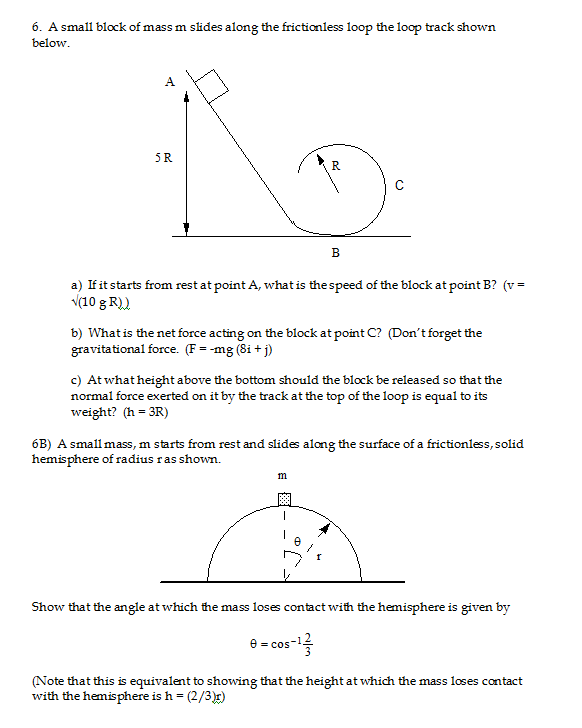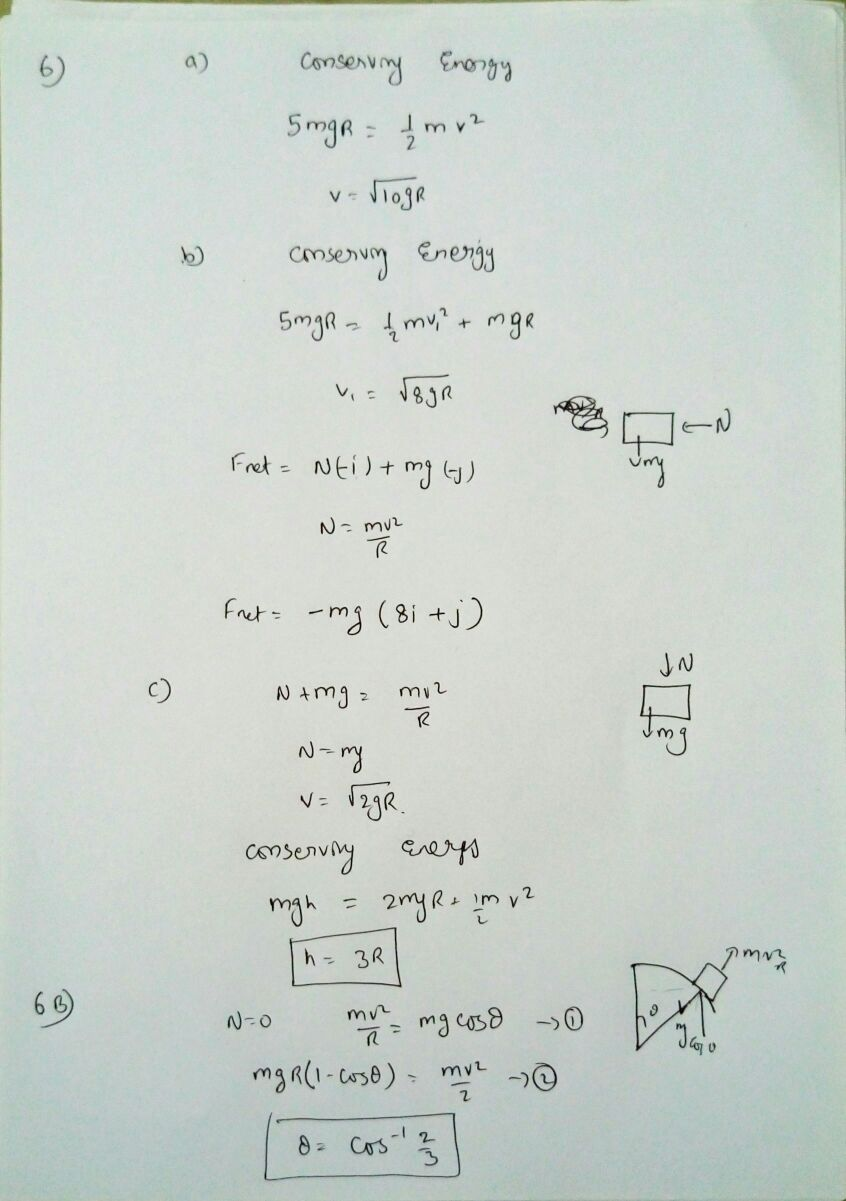#### Earn Coins

Coins can be redeemed for fabulous gifts.

Similar Homework Help Questions
• ### A block of mass m slides down a frictionless ramp to a loop of radius R,...A block of mass m slides down a frictionless ramp to a loop of radius R, also frictionless. It's initial height above the bottom of the loop is ?h = 3R and the block starts at rest. What is the magnitude of the normal force that the track of the loop exerts on the block at position 2? You can assume that the block is exactly at the horizontal height of the center of the loop when it reaches position...

• ### A block starts from rest and slides without friction along the surface of a hemisphere of...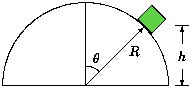A block starts from rest and slides without friction along the surface of a hemisphere of radius R = 1.7 m. As the block slides, eventually it loses contact with the hemisphere. What is the height, h, when the block loses contact with the hemisphere? A block starts from rest and slides without friction along the surface of a hemisphere of radius R = 1.7 m. As the block slides, eventually it loses contact with the hemisphere.What is the height,...

• ### As shown in the figure below, a block of mass m starts from rest and slides...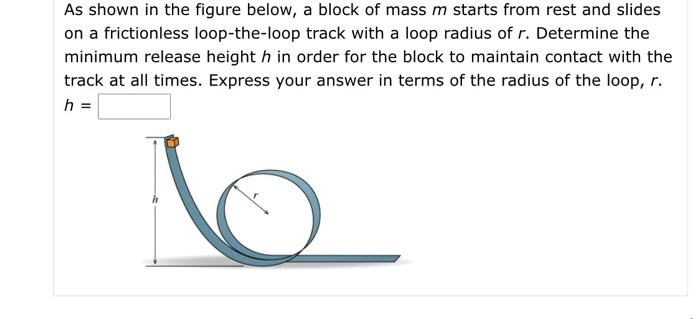As shown in the figure below, a block of mass m starts from rest and slides on a frictionless loop-the-loop track with a loop radius of r. Determine the minimum release height h in order for the block to maintain contact with the track at all times. Express your answer in terms of the radius of the loop, r. h = lo

• ### Loop the Loop Figure 1)A roller coaster car may be approximated by a block of mass...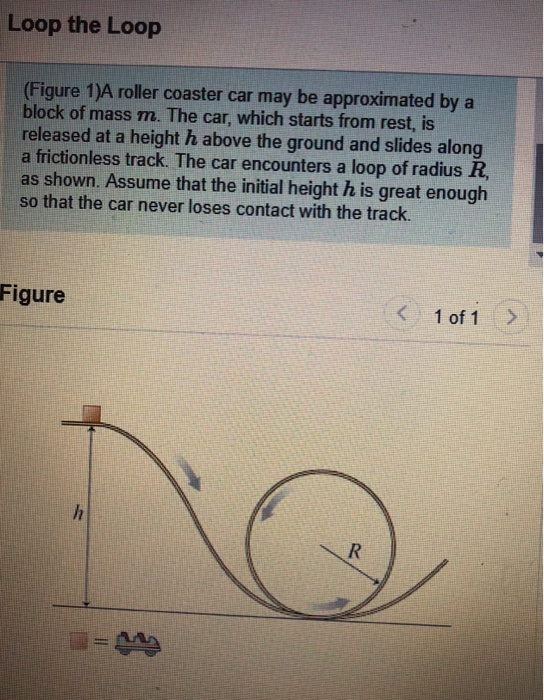Loop the Loop Figure 1)A roller coaster car may be approximated by a block of mass m. The car, which starts from rest, is released at a height h above the ground and slides along a frictionless track. The car encounters a loop of radius R as shown. Assume that the initial height h is great enough so that the car never loses contact with the track. Figure 1 of 1 위부

• ### In the figure, a small block of mass m = 0.022 kg can slide along the...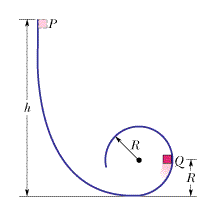In the figure, a small block of mass m = 0.022 kg can slide along the frictionless loop-the-loop, with loop radius R = 15 cm. The block is released from rest at point P, at height h = 5R above the bottom of the loop. What are the magnitudes of (a) the horizontal component and (b) the vertical component of the net force acting on the block at point Q? (c) At what height h should the block be released...

• ### A box slides from rest down a frictionless ramp inclined at 38.0° with respect to the...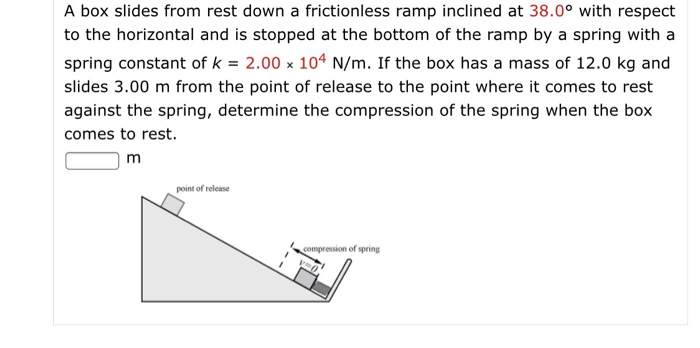A box slides from rest down a frictionless ramp inclined at 38.0° with respect to the horizontal and is stopped at the bottom of the ramp by a spring with a spring constant of k = 2.00 x 104 N/m. If the box has a mass of 12.0 kg and slides 3.00 m from the point of release to the point where it comes to rest against the spring, determine the compression of the spring when the box comes to...

• ### 1. Ice cube in a loop A small ice cube of mass m slides on a...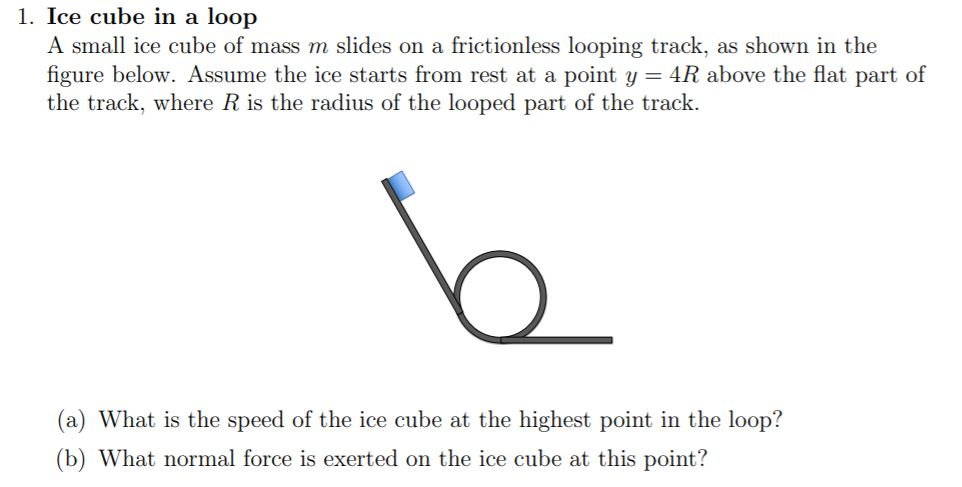1. Ice cube in a loop A small ice cube of mass m slides on a frictionless looping track, as shown in the figure below. Assume the ice starts from rest at a point y = 4R above the flat part of the track, where R is the radius of the looped part of the track. (a) What is the speed of the ice cube at the highest point in the loop? (b) What normal force is exerted on the...

• ### In the figure, a small block of mass m = 0.040 kg can slide along the...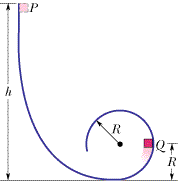In the figure, a small block of mass m = 0.040 kg can slide along the frictionless loop-the-loop, with loop radius R = 19 cm. The block is released from rest at point P, at height h = 6R above the bottom of the loop. How much work does the gravitational force do on the block as the block travels from point P to (a) point Q and (b) the top of the loop? If the gravitational potential energy of...

• ### A block of mass m slides down a frictionless incline. The block is released a height...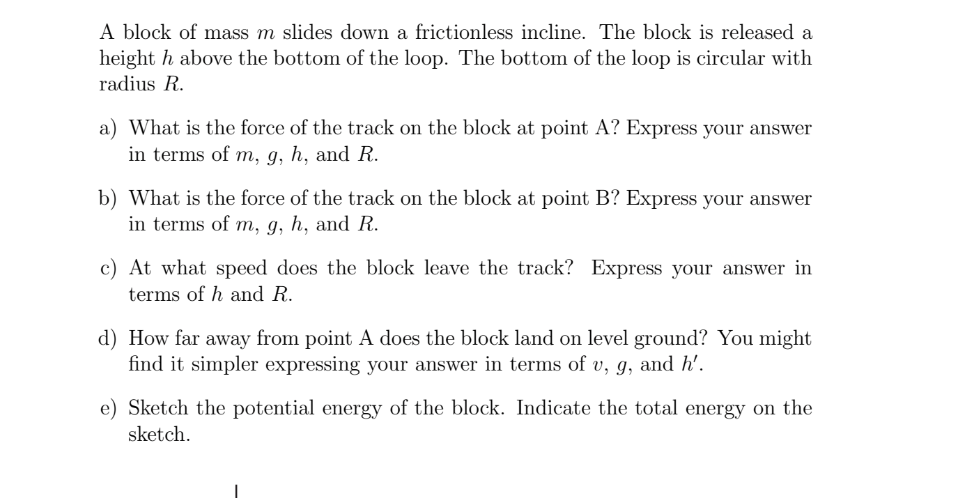A block of mass m slides down a frictionless incline. The block is released a height h above the bottom of the loop. The bottom of the loop is circular with radius R. a) What is the force of the track on the block at point A? Express your answer in terms of m, g, h, and R. b) What is the force of the track on the block at point B? Express your answer in terms of m, g,...

• ### Problem 3 A block of mass m slides down a frictionless incline. The block is released...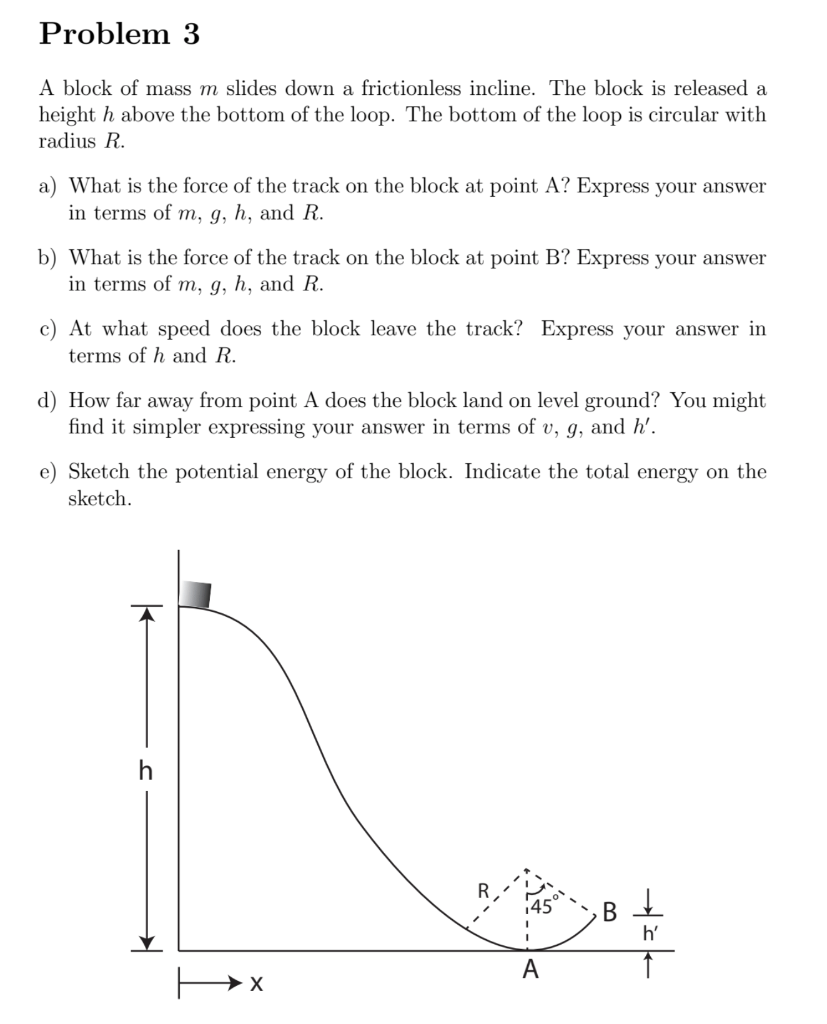Problem 3 A block of mass m slides down a frictionless incline. The block is released a height h above the bottom of the loop. The bottom of the loop is circular with radius R. a) What is the force of the track on the block at point A? Express your answer in terms of m, g, h, and R. b) What is the force of the track on the block at point B? Express your answer in terms of...